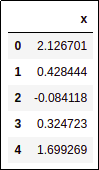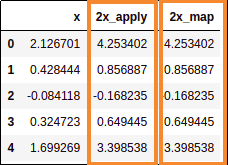Pandas for Large Data: Examples and Tips

# Pandas for Large Data: Examples and Tips

Last updated:

WIP Alert This is a work in progress. Current information is correct but more content may be added in the future.

## Use small data types

Use df.dtypes to see what your dataframe dtypes look like

Pandas sometimes defaults to unnecessarily large datatypes.

Use the smallest dtypes you possibly can such as:

• 'uint8' instead of 'int32' or 'int64'

• 'float32' instead of 'float64'

• 'category' instead 'object' for categorical data

## Delete objects from memory

Delete DataFrames and other variables you will not use anymore using:

• del(my_df) deletes the reference to your variable

• gc.collect() explictly frees memory space.

Example:

import gc
import pandas as pd

df1_processed = process_df(df1)

# df1 is not needed anymore
del(df1)
gc.collect()

# you'll have more memory to load other dataframes
// ...


## Do joins chunk by chunk

Perform large, complex joins in parts to avoid exploding memory usage:

# split dataframe indices into parts
indexes = np.linspace(0, len(second_df), num=10, dtype=np.int32)

# update in small portions
for i in range(len(indexes)-1):
my_df = pd.concat(
[
my_df, # the same DF
pd.merge(left=pd.merge(
left=second_df.loc[indexes[i]:indexes[i+1], :],
right=third_df,
how='left',
on='foreign_key'
),
right=fourth_df,
how='left',
on='other_foreign_key'
)
]
)


## Use vectorized numpy functions when possible

For example, use DataFrame.apply instead of Series.map to create derived columns and/or to operate on columns:

Time comparison: create a dataframe with 10,000,000 rows and multiply a numeric column by 2

import pandas as pd
import numpy as np

# create a sample dataframe with 10,000,000 rows
df = pd.DataFrame({
'x': np.random.normal(loc=0.0, scale=1.0, size=10000000)
})Sample dataframe for benchmarking
(top 5 rows shown only)

Using map function multiply 'x' column by 2

def multiply_by_two_map(x):
return x*2

df['2x_map'] = df['x'].map(multiply_by_two)
# >>> CPU times: user 14.4 s, sys: 300 ms, total: 14.7 s
# >>> Wall time: 14.7 s


Using apply function multiply 'x' column by 2

import numpy as np

def multiply_by_two(arr):
return np.multiply(arr,2)

# note the double square brackets around the 'x'!!
# this is because we want to use DataFrame.apply,
# not Series.apply!!
df['2x_apply'] = df[['x']].apply(multiply_by_two)
# >>> CPU times: user 80 ms, sys: 112 ms, total: 192 ms
# >>> Wall time: 188 msBoth map and apply yielded the same results
but the apply version was around
70 times faster!

## Parallel apply with swifter

see pandas column operations: map vs apply for a comparison between map and apply

The full comparison code is on this notebook

Swifter is a library that aims to parallelize Pandas apply whenever possible.

It is not always the case that using swifter is faster than a simple Series.

Dataframe.apply Series.apply Dataframe.apply (Swifter) Series.apply (swifter)
Vectorizable operations0.5 secs6 secs0.3 secs0.2 secs
Number to string24 secs137 secs
Simple if-then-else4.5 secs3 secs

## Parallel dataframe processing with multiprocessing

All chunks must fit in memory at the same time!

Spawn multiple Python processes and have each of them process a chunk of a large dataframe.

This will reduce the processing time by half or even more, depending on the number of processe you use.

All code available on this jupyter notebook

Example: use 8 cores to process a text dataframe in parallel.

• Step 1: put all processing logic into a single function:

import pandas as pd

# this is an example of common operations you have to perform on text
# data to go from raw text to clean text you can use for modelling
def process_df_function(df):

output_df = df.copy()

# replace weird double quotes with normal ones
output_df['text']      = output_df['text'].apply(lambda text: text.replace("",'"'))

# text to lower case
output_df['text']      = output_df['text'].apply(lambda text: text.lower())

# replace number with a special token
output_df['text']      = output_df['text'].apply(lambda text: re.sub(r"\b\d+\b","tok_num", text))

# get the number of words in each text
output_df['num_words'] = output_df['text'].apply(lambda text: len(re.split(r"(?:\s+)|(?:,)|(?:\-)",text)))

# take out texts that are too large
indices_to_remove_too_large = output_df[output_df['num_words'] > 50]
output_df.drop(indices_to_remove_too_large.index, inplace=True)

# or too small
indices_to_remove_too_small = output_df[output_df['num_words'] < 10]
output_df.drop(indices_to_remove_too_small.index, inplace=True)

output_df.reset_index(drop=True, inplace=True)

return output_df

• Step 2: Use multiprocessing.Pool to distribute the work over multiple processes

import multiprocessing
import numpy as np
import pandas as pd

# load raw data into a dataframe

NUM_CORES = 8

# split the raw dataframe into chunks
df_chunks = np.array_split(raw_df ,NUM_CORES)

# use a pool to spawn multiple proecsses
with multiprocessing.Pool(NUM_CORES) as pool:

# concatenate all processed chunks together.
# process_df_function is the function you defined in the previous block
processed_df = pd.concat(pool.map(process_df_function, df_chunks), ignore_index=True)